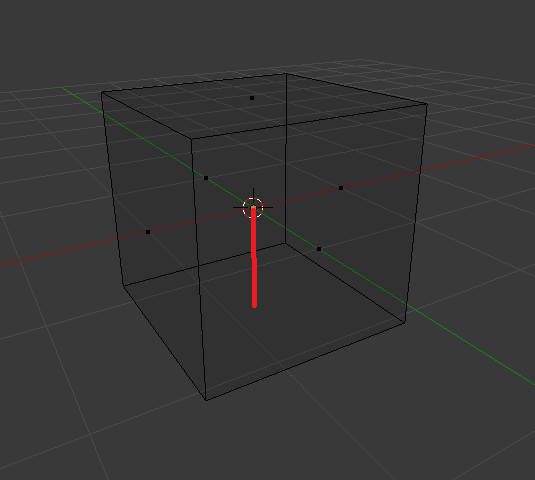# Calculate distance from object origin to edge

How do I calculate the distance from an objects origin to the lowest point on the z axis?
Or : Distance between object origin and median point of objects lower face.

To clarify, the red line in the image below:Thanks alot

(Relatively new to python)2nd example

This is not so much an engine thing but general math. Now, pythagoras says `sqrt( ((p0.x-p1.x)**2) + ((p0.y-p1.y)**2) )` but of course you’d need to know the coordinates of the points. Do you?

Calculating via coordinates would only apply to a static mesh right? I want to create a python script that I can use universally on different, more complex objects no matter their world location.

I first tried with using a ray-sensor pointing straight down, but im not sure how to get a hitposition where the object ends. The ray only detects hitposition of other objects?

Like this? You can easily only set axis positions with .x, .y, or .z values at the end of either position function.
Or are you looking for a lerp calculation instead?

``````KX_GameObject.worldPosition - KX_GameObject.localPosition
``````

A coordinate is a point in space. Moving or not doesn’t matter because the distance between points remains constant.

If you scale the model however, it will change.
And if you rotate the model, the lowest point in z may also change.

But you don’t know where this point is, so that’s the more immediate problem. Getting the median of a quad requires a little bit more fiddling so that’d be your homework; look into mesh polygons for that.

Here is an approximation going by lowest points, only because it is more convenient and easier for me to explain.

``````from bge.logic import getCurrentController;
from mathutils import Vector;
own=getCurrentController().owner; me=own.meshes;

low_point=Vector([0,0,0]); points=[];
for matid in range(me.numMaterials):
for vi in range(me.getVertexArrayLength(matid)):
vert=me.getVertex(matid, vi); co=vert.XYZ;
co=(Vector([co.x, co.y, co.z, 1])*own.worldTransform).xyz;
if co.z < low_point.z:
low_point=co;

if co not in points: points.append(co);

points=[co for co in points if co.z==low_point.z];
low_point=Vector([0,0,0]);
for co in points:
low_point+=co;

low_point=low_point/len(points);
low_point.xy = -low_point.xy;

dist=low_point.length;

``````

What goes on in here is I iterate through each vertex in the mesh, move them around according to object transforms, compare the z values and get the lowest. Finally, I take the median XY point between all points whose Z is equal to the lowest point, giving you something close to the center point of the lowest edge.

Finally, since all vertex positions are relative to the origin, the length of the resulting vector should be equal or at least fairly close to the distance you want.

For testing, I made a red icosphere snap to the final position of `low_point` and made a cuboid rotate along X and Y. Here’s what that looks like:

Do note that this isn’t very performant for real time, and worse still on complex meshes. Your best bet might be caching the results for later use rather than calculating them on the spot.

Have you tried raycasting from another object (like an Empty) positioned at your object’s origin?

``````from bge import logic
from mathutils import Vector

Cube = logic.getCurrentController().owner

scene = logic.getCurrentScene()

Empty = scene.objects['Empty']
Empty.worldPosition = Cube.worldPosition

range = 500

prop = 'Cube' # the Cube must have a property (of any kind) named 'Cube'

facenormal = 1

xray = 1 # skip objects that don’t match prop

object, hitpoint, hitnormal = Empty.rayCast(Vector([0.0, 0.0, -range]), Cube.worldPosition, range, prop, facenormal, xray)

print(object) # it should always be the Cube
print(hitpoint)
print(hitnormal)
``````